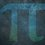# UKMT Special (Problem $5$)

The integers $a, b, c, d, e, f, g$, none of which is negative, satisfy the following system of simultaneous equations:

$a + b + c = 2$

$b + c + d = 2$

$c + d + e = 2$

$d + e + f = 2$

$e + f + g = 2$

Find the maximum possible value of $a + b + c + d + e + f + g$

[UKMT Cayley Olympiad $2015$, Q$2$]Note by Yajat Shamji
8 months, 2 weeks ago

This discussion board is a place to discuss our Daily Challenges and the math and science related to those challenges. Explanations are more than just a solution — they should explain the steps and thinking strategies that you used to obtain the solution. Comments should further the discussion of math and science.

When posting on Brilliant:

• Use the emojis to react to an explanation, whether you're congratulating a job well done , or just really confused .
• Ask specific questions about the challenge or the steps in somebody's explanation. Well-posed questions can add a lot to the discussion, but posting "I don't understand!" doesn't help anyone.
• Try to contribute something new to the discussion, whether it is an extension, generalization or other idea related to the challenge.

MarkdownAppears as
*italics* or _italics_ italics
**bold** or __bold__ bold
- bulleted- list
• bulleted
• list
1. numbered2. list
1. numbered
2. list
Note: you must add a full line of space before and after lists for them to show up correctly
paragraph 1paragraph 2

paragraph 1

paragraph 2

[example link](https://brilliant.org)example link
> This is a quote
This is a quote
    # I indented these lines
# 4 spaces, and now they show
# up as a code block.

print "hello world"
# I indented these lines
# 4 spaces, and now they show
# up as a code block.

print "hello world"
MathAppears as
Remember to wrap math in $$ ... $$ or $ ... $ to ensure proper formatting.
2 \times 3 $2 \times 3$
2^{34} $2^{34}$
a_{i-1} $a_{i-1}$
\frac{2}{3} $\frac{2}{3}$
\sqrt{2} $\sqrt{2}$
\sum_{i=1}^3 $\sum_{i=1}^3$
\sin \theta $\sin \theta$
\boxed{123} $\boxed{123}$

Sort by:

You have until this Sunday, $3:00$pm!

- 8 months, 2 weeks ago

As all the equations are equal to the same thing, we know they must be equal by Euclid's Axiom (Things which are equal to the same thing are also equal to one another)

$a+b+c=b+c+d=c+d+e=d+e+f=e+f+g$

From the above equations, we can simplify to get $\to a=d=g,$ $b=e$ and $c=f$

So the equation we want to find the maximum possible value of gets simplified

$a+b+c+d+e+f+g=a+b+c+a+b+c+a=2(a+b+c)+a=4+a$

We know that a can't be lesser than $0$ or greater than $2$, and that it must be an integer. This leaves the possible options of $0, 1$ and $2$. All of these options will work, but $2$ is the greatest value here.

Thus, the maximum value of the equation is $\boxed{6}$

- 8 months, 2 weeks ago

@Yajat Shamji - Done :)

- 8 months, 2 weeks ago

Correct!

But... different method.

- 8 months, 2 weeks ago

Yet again I see...

- 8 months, 2 weeks ago